# Physical Characterization of Materials Using Porosimetry

Mercury intrusion porosimetry is one of only a few analytical techniques that permits an analyst to acquire data over such a broad dynamic range using a single theoretical model. Mercury porosimetry routinely is applied over a capillary diameter range from 0.003 µm to 360 µm— five orders of magnitude! This is equivalent to using the same tool to measure with accuracy and precision the diameter of a grain of sand and the height of a 30-story building.

Not only is mercury porosimetry applicable over a wide range of pore sizes, but also the fundamental data it produces (the volume of mercury intruded into the sample as a function of applied pressure) is indicative of various characteristics of the pore space and is used to reveal a variety of physical properties of the solid material itself.

The information that follows falls into three main categories: I) instrument theory and its application in data collection, II) information derived from reduced data, and III) presentation of the information. A glossary of terms also is included.

Understanding how a fluid behaves under specific conditions provides insight into exactly how a mercury porosimeter probes the surface of a material and moves within the pore structure. This allows one to better understand what mercury intrusion and extrusion data mean in relation to the sample under test and allows one to understand the data outside of the bounds of the theoretical model. It also allows one to make an educated comparison between similar data obtained using other measurement techniques and theoretical models.

The information contained herein pertains for the most part to the general technique of mercury porosimetry without regard to a specific instrument manufacturer or model. However, Micromeritics’ AutoPore series of porosimeters is used as a reference, particularly when examples are required and details of data reduction are presented.

## Theory and Method of Measurement

Routine operation of an analytical instrument does not require knowledge of the fundamentals of instrument theory. However, an in-depth understanding of the relationship between the probe and the sample allows one to interpret data outside of the strict limitations of the theoretical model upon which data reduction is based. Although this may have limited relevance for day-to-day quality or process control applications, it is of extreme importance in research work and when developing analysis methods for control applications. For these reasons, this document begins with information about how a nonwetting liquid (specifically, mercury) reacts in seeking equilibrium between internal and external forces at the liquid-solid, liquid-vapor, and liquidsolid-vapor interfaces.

## Fluid Dynamics and Capillary Hydrostatics

Consider a drop of liquid resting on a solid surface as shown in Figure 1. The underside of the liquid is in contact with the solid surface. The remainder of the surface of the liquid is in contact with some other fluid above— typically, either its own vapor or air. In this configuration, there are areas of liquid-solid, liquid-vapor, and solid-vapor interfaces. There also exists a liquid-solid-vapor boundary described by a line.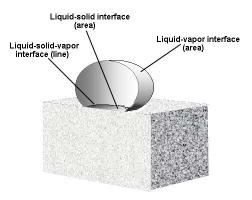Figure 1. Cross-section of a drop of non-wetting liquid resting on a solid surface. All interfaces are shown.

There is tension in each interface. The liquidvapor interfacial tension is symbolized gl-v, the liquid liquid-solid gl-s, and the solid-vapor gs- v. The liquid-vapor and solid-vapor interfacial tensions also are referred to as surface tensions. Surface tension has dimensions of force per unit length and acts tangentially to the interface.

The angle of contact of the liquid-vapor surface to the solid-vapor surface at a point on the liquid-solidvapor interface characterizes the interfacial tension present between the solid, liquid, and vapor. Figure 2 shows five liquids of different surface tensions resting on the same surface material. Different surface energies cause the liquids to assume different contact angles relative to the solid surface.

A liquid with low surface tension (low surface energy) resting on a solid surface of higher surface tension will spread out on the surface forming a contact angle less than 90°; this is referred to as wetting. If the surface energy of the liquid exceeds that of the solid, the liquid will form a bead and the angle of contact will be between 90° and 180°; this is a non-wetting liquid relative to the surface.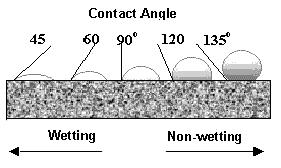Figure 2. Various liquids resting on a solid surface. The different angles of contact are illustrated for wetting and non-wetting liquids.

Considering any point along the line that describes the liquid-solid-vapor interface and indicating all force vectors on that point results in a diagram similar to those of Figure 3. These illustrations represent a time sequence (top to bottom) showing what happens when a liquid drop first is placed on a horizontal surface until it achieves equilibrium. One can imagine the initial, somewhat spherical drop flattening and spreading over the surface prior to stabilizing.

The contact angle begins at about 180°, and the liquid–vapor tension vector at the liquidsolid- vapor interface points at the angle of contact. As the contact angle decreases, the horizontal component of the liquid-vapor tension vector changes in magnitude and, if the contact angle decreases past 90°, the horizontal component changes sign. When the sum of the solid-vapor tension vector, liquid-solid tension vector, and horizontal component of the liquid-vapor tension vector equal zero, equilibrium occurs and spreading ceases.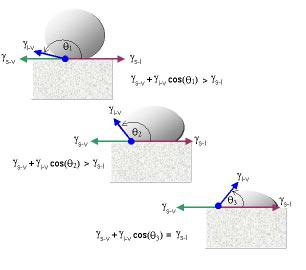Figure 3. A droplet of liquid placed on a solid surface assumes a contact angle that balances the horizontal force components of the three tension vectors. For this example, è3 is the angle that results in equilibrium.

The surface of the liquid at the liquid-vapor interface assumes a curvature having two radii, r1 and r2, one in the x-z plane, the other in the y-z plane, where the solid surface is the x-y plane. This is another effect of surface tension. The surface molecules act like an elastic membrane pulling the surface into the smallest configuration, ideally a sphere where r1 = r2 = r. Surface tension contracts the surface and volume until the internal force Fi per unit area of surface A2 is in equilibrium with the external forces on the same surface element. Since pressure, P, is force per unit area (F/A), equilibrium can be expressed in terms of internal and external pressures. From the equations of Young and Laplace for spherical surfaces, the difference in pressure across the surface is

P” - P’ = ϒ (1/r1 + 1/r2) = 2ϒ /r     (1)

where P” is the pressure on the concave side, P’ the pressure on the convex side, g the liquid-vapor surface tension, and, since it is a spherical surface, r1 = r2.

Interfacial tensions also cause liquids to exhibit capillarity. If one end of a capillary tube is forced to penetrate the vapor-liquid surface from the vapor side, a wetting liquid spontaneously enters the capillary and rises to a level above the external liquidvapor interface. A non-wetting liquid resists entering the capillary and that a level always below the external liquid-vapor level. In other words, a nonwetting liquid must be forced to enter a capillary.

Why does a non-wetting liquid resist entry into a capillary? Inside the capillary and along the line describing the vapor-liquid-solid boundary, the liquid-solid interface assumes an angle that results in equilibrium of forces. The contributing forces are those of cohesion between the liquid molecules, and the force of adhesion between the liquid molecules and the walls of the capillary.

The liquid-vapor interface in the capillary (the meniscus) is concave for a wetting liquid and convex for a non-wetting liquid. In summary, there are three physical parameters needed to describe the intrusion of a liquid into a capillary: a) the interfacial tension (surface tension) of the liquid-vapor interface, hereafter symbolized simply by g, b) the contact angle q, and c) the geometry of the line of contact at the solid -liquid-vapor boundary. For a circular line of contact, the geometry is described by pr2, where r is the radius of the circle or capillary.

Washburn in 1921 derived an equation describing the equilibrium of the internal and external forces on the liquid-solid-vapor system in terms of these three parameters. It states concisely that the pressure required to force a non-wetting liquid to enter a capillary of circular cross-section is inversely proportional to the diameter of the capillary and directly proportional to the surface tension of the liquid and the angle of contact with the solid surface. This physical principal was incorporated into an intrusion- based, pore-measuring instrument by Ritter & Drake in 1945. Mercury is used almost exclusively as the liquid of choice for intrusion porosimetry because it is non-wetting to most solid materials.

Washburn’s equation, upon which data reduction is based, assumes that the pore or capillary is cylindrical and the opening is circular in cross-section. As has been stated, the net force tends to resist entry of the mercury into the pore and this force is applied along the line of contact of the mercury, solid, and (mercury) vapor. The line of contact has a length of 2pr and the component of force pushing the mercury out of the capillary acts in the direction cosq (see Figure 4), where q is the liquid-solid contact angle. The magnitude of force tending to expel the mercury is

FE = 2 π r ϒ cos θ     (2)

where ϒ is the surface tension.

An external pressure on the mercury is required to force its entry into the pore. The relationship between force (F) and pressure (P) is P = F/area. Solving for force gives

FI = π r2P     (3)

Where π r2 is the cross-sectional area of the pore opening.

Balancing the intrusion and extrusion forces results in the Washburn equation

-2 πr ϒ cos θ = πr2P      (3)

or, in terms of diameter D,

-πD ϒ cos θ = ( π D2P)/4     (4)

The relationship between applied pressure and the minimum size pore into which mercury will be forced to enter is

D = -4 ϒ cos θ/P (5)

For a given liquid-solid system, the numerator is constant, providing the simple relationship expressing that the size of the pore into which mercury will intrude is inversely proportional to the applied pressure. In other words, mercury under external pressure P can resist entry into pores smaller than D, but cannot resist entry into pores of sizes larger than D. So, for any pressure, it can be determined which pore sizes have been invaded with mercury and which sizes have not.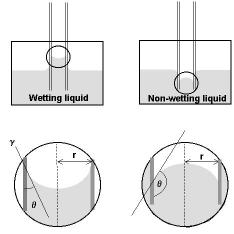Figure 4. Capillary action of a wetting and non-wetting liquid relative to the walls of a capillary. The g indicates the direction of the interfacial tension (force) vector.

## Collecting Experimental Data

A typical mercury intrusion porosimetry test involves placing a sample into a container, evacuating the container to remove contaminant gases and vapors (usually water) and, while still evacuated, allowing mercury to fill the container. This creates an environment consisting of a solid, a non-wetting liquid (mercury), and mercury vapor. Next, pressure is increased toward ambient while the volume of mercury entering larger openings in the sample bulk is monitored. When pressure has returned to ambient, pores of diameters down to about 12 mm have been filled.

The sample container is then placed in a pressure vessel for the remainder of the test. A maximum pressure of about 60,000 psia (414 MPa) is typical for commercial instruments and this pressure will force mercury into pores down to about 0.003 micrometers in diameter. The volume of mercury that intrudes into the sample due to an increase in pressure from Pi to Pi+1 is equal to the volume of the pores in the associated size range ri to ri+1, sizes being determined by substituting pressure values into Washburn’s equation, Eq. 5.

The measurement of the volume of mercury moving into the sample may be accomplished in various ways. A common method that provides high sensitivity is to attach a capillary tube to the sample cup and allow the capillary tube to be the reservoir for mercury during the experiment. Only a small volume of mercury is required to produce a long ‘string’ of mercury in a small capillary. When external pressure changes, the variation in the length of the mercury column in the capillary indicates the volume passing into or out of the sample cup.

For example, a capillary of 1 mm radius requires only 0.03 cm3 of mercury to produce a mercury column 1 mm in length. Therefore, volume resolution of 0.003 cm3 easily could be obtained visually from a scale etched on the capillary stem. However, electronic means of detecting the rise and fall of mercury within the capillary are much more sensitive, providing even greater volume sensitivity down to less than a microliter. The measurement of a series of applied pressures and the cumulative volumes of mercury intruded at each pressure comprises the raw data set. A plot of these data is called the intrusion curve. When pressure is reduced, mercury leaves the pores, or extrudes. This process also is monitored and plotted and is the extrusion curve. According to the shape of the pores and other physical phenomena, the extrusion curve usually does not follow the same plotted path as the intrusion curve. Therefore, the intrusion curve and extrusion curve contain different information about the pore network.

When to collect the data point is an important consideration when measuring intrusion and extrusion characteristics. Since the intrusion process involves moving a mass of mercury into a confined pore space, the process is not instantaneous as exemplified by the Hagen- Poiseuille law

Q = V/t = (πr4/8 η)(ΔP/l)      (6)

where Q = flow of the liquid, V the volume of liquid, t time, r the capillary radius, η the liquid viscosity and ΔP/l the pressure drop per unit length of capillary.

However, long and tortuous pore channels result in smaller Q values, therefore requiring more time to fill the same volume as would be the case for pore systems having higher Q values. To obtain highly resolved and highly accurate data, the intrusion process must be allowed to equilibrate before changing pressure and probing the next smaller-sized pore class. Expressed another way, high-resolution data collection, particularly in the small pore size range, requires a pressure step, that is, pressure is raised to the next pressure, then held until flow ceases. Scanning mode, in which pressure is continually changed, is best employed for very large pores or for screening purposes.

## Measurement Transducers

From the above discussion, it is clear that a mercury porosimeter measures only applied pressure and the volume of mercury intruded into or extruded from the sample bulk. Pressure measurements are obtained by pressure transducers that produce an electrical signal (current or voltage) that is proportional to the amplitude of the pressure applied to the sensor. This analog electrical signal is converted into digital code for processing by the monitoring computer.

The transducer that detects mercury volume is integrated into the sample holder assembly as previously exemplified and shown in Figure 5.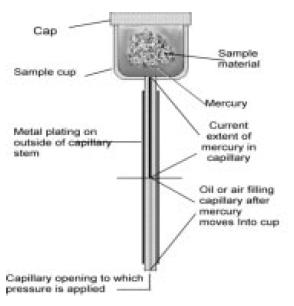Figure 5. Cross-section of a penetrometer in which pressure has forced some mercury into the pores of the sample and about 50% of the stem capacity has been used.

The sample cup has a capillary stem attached and this capillary serves both as the mercury reservoir during analysis and as an element of the mercury volume transducer. Prior to the beginning of each analysis, the sample cup and capillary are filled with mercury. After filling, the main source of mercury is removed leaving only the mercury in the sample cup and capillary stem, the combination being referred to as the penetrometer. Pressure is applied to the mercury in the capillary either by a gas (air) or a liquid (oil). The pressure is transmitted from the far end of the capillary to the mercury surrounding the sample in the sample cup.

The capillary stem is constructed of glass (an electrical insulator), is filled with mercury (an electrical conductor), and the outer surface of the capillary stem is plated with metal (an electrical conductor). The combination of two concentric electrical conductors separated by an insulator produces a co-axial capacitor. The value of the capacitance is a function of the areas of the conductors, the dielectric constant of the insulator, and other physical parameters. In the case of this particular capacitor, the only variable is the area of the interior conductor as mercury leaves the capillary and enters the sample voids and pores, or as it moves back into the capillary when pressure is reduced.

This is mechanically analogous to a mercury thermometer in which case mercury moves in and out of a calibrated capillary from a large bulb at one end. A small volume of mercury entering or leaving a small capillary causes the length (and area) of the mercury column to change significantly, thus providing volume-measuring sensitivity and resolution. In the case of the thermometer, the change in volume is proportional to the change in temperature by the coefficient of volumetric expansion of mercury.

The capacitance value of the stem is monitored by a capacitance detector that, similar to the pressure transducer electronics, produces an electrical signal that is proportional to capacitance. Capacitance measurements are transformed into volume measurements by knowledge of the diameter of the precision capillary and the equation governing coaxial capacitors.

##This information has been sourced, reviewed and adapted from materials provided by Micromeritics Instrument Corporation.

## Citations

• APA

Micromeritics Instrument Corporation. (2019, December 06). Physical Characterization of Materials Using Porosimetry. AZoNano. Retrieved on July 05, 2020 from https://www.azonano.com/article.aspx?ArticleID=2641.

• MLA

Micromeritics Instrument Corporation. "Physical Characterization of Materials Using Porosimetry". AZoNano. 05 July 2020. <https://www.azonano.com/article.aspx?ArticleID=2641>.

• Chicago

Micromeritics Instrument Corporation. "Physical Characterization of Materials Using Porosimetry". AZoNano. https://www.azonano.com/article.aspx?ArticleID=2641. (accessed July 05, 2020).

• Harvard

Micromeritics Instrument Corporation. 2019. Physical Characterization of Materials Using Porosimetry. AZoNano, viewed 05 July 2020, https://www.azonano.com/article.aspx?ArticleID=2641.